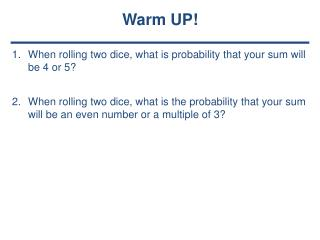# When rolling two dice, what is probability that your sum will be 4 or 5 ? - PowerPoint PPT PresentationDownload PresentationWhen rolling two dice, what is probability that your sum will be 4 or 5 ?

When rolling two dice, what is probability that your sum will be 4 or 5 ?Download Presentation## When rolling two dice, what is probability that your sum will be 4 or 5 ?

- - - - - - - - - - - - - - - - - - - - - - - - - - - E N D - - - - - - - - - - - - - - - - - - - - - - - - - - -
##### Presentation Transcript

1. Warm UP! When rolling two dice, what is probability that your sum will be 4 or 5? When rolling two dice, what is the probability that your sum will be an even number or a multiple of 3?

2. Mutually Exclusive Events • Example 3: • What is the probability of picking a queen or an ace from a deck of cards

3. Overlapping Events • Probability that overlapping events A and B or both will occur expressed as: • P(M or E) = P(M) + P(E) - P(ME)

4. Overlapping Events • Example 1: • Find the probability of picking a king or a club in a deck of cards. 4/13

5. Overlapping Events • Example 2: • Find the probability of picking a female or a person from Tennessee out of the committee members.

6. Overlapping Events • Example 3: • When rolling 2 dice, what is the probability of getting an even sum or a number greater than 10?

7. You Try… • 100 people were asked their favorite fast food restaurant. The table below has the information from the survey: • What is the probability that a person likes Wendy’s? • What is the probability that a person is male given they like Burger King? • 3. What is the probability that a person is female or likes McDonald’s? 7/20 3/5 3/4

8. What’s the difference?

9. Independent Events

10. Experiment 1 A jar contains 3 red, 5 green, 2 blue and 6 yellow marbles. A marble is chosen at random from the jar. After replacing it, a second marble is chosen. What is the probability of choosing a green and a yellow marble?

11. Dependent Events

12. Experiment 2 A random sample of parts coming off a machine is done by an inspector. He found that 5 out of 100 parts are bad on average. If he were to do a new sample, what is the probability that he picks a bad part and then picks another bad part if he doesn’t replace the first?

13. HOT SEAT! Please feel under your chair. If you have a sticky note (and I know what seats do… there are 4 sticky notes) you are the LUCKY ONES today! \$600 \$400 Bankrupt \$200 \$500 Bankrupt \$300 \$700

14. Class Work • Complete the worksheet with a partner • It is due at the end of class (for a grade)

15. TOTD Answer the following 2-part question before you leave: • A basket of apples contains 11 apples – 6 are red, 2 are green, and 3 are yellow. You randomly select 2 apples, one at a time. Find the probability that both are yellow if • You replace the first apple, then select the second • You eat the first apple, then select the second.

16. Homework: Pg. 354 1 - 8